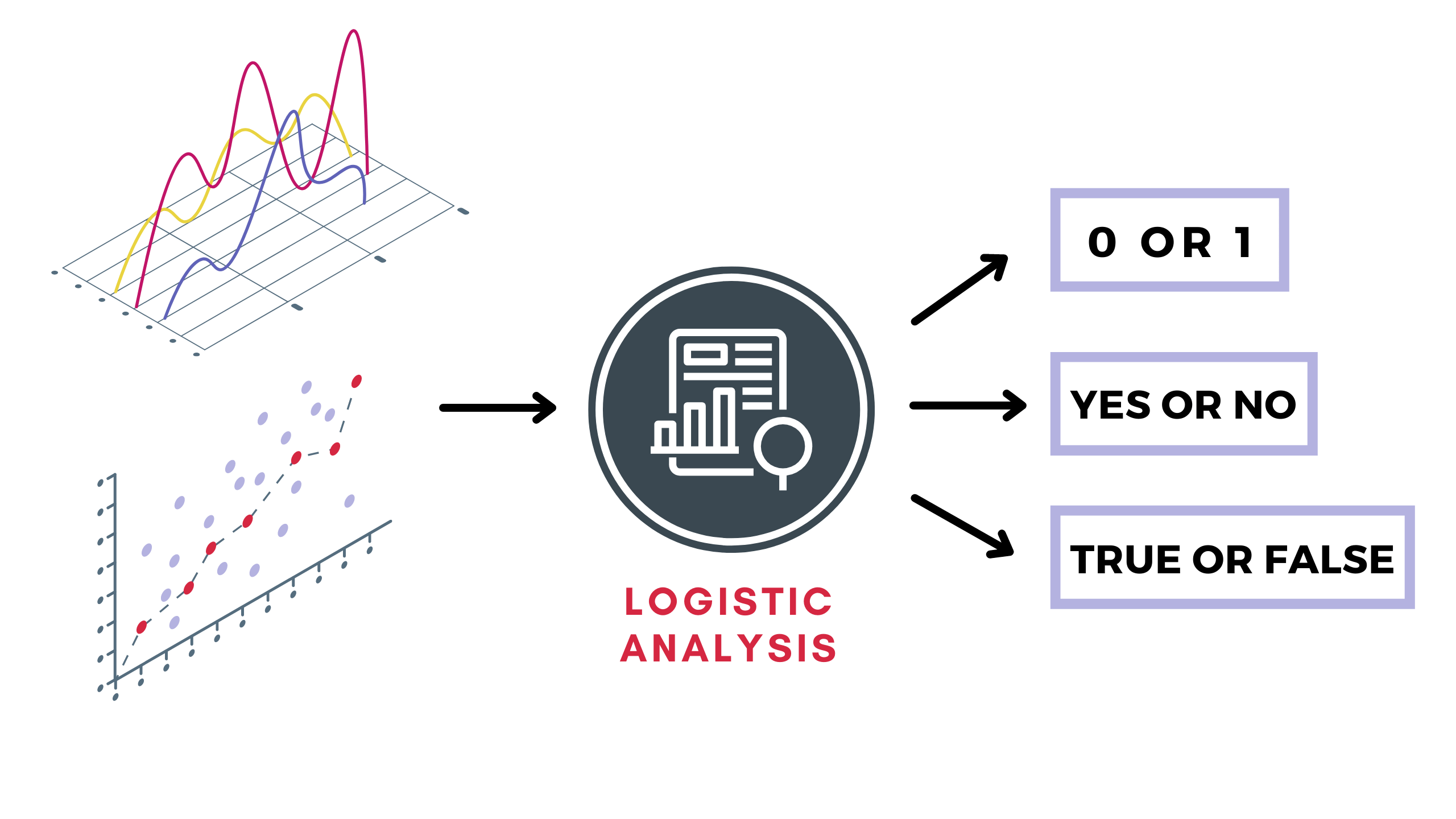In this section, we are going to start with another Supervised Learning Technique of Regression that is, Logistic Regression! It is much similar to the last technique we learned, Linear Regression except for the fact that Logistic Regression is used to solve classification problems. Let’s learn more about it.

Logistic Regression predicts the outcome of a categorical dependent variable in a binary format. Thus the result should be categorical/discrete such as:The above diagram is termed Binary Classification. Another type of classification may differ from the outcome in the analysis above. Let’s consider there is a classification problem with three options to choose from instead of two. It will be termed Multiclass classification. For example, you have data of the weights of people split into categories of not obese and obese. This means on the basis of weight, people are categorized into obese and not obese.

Let’s consider a data set for employees of a company having insurance. This will give us in-depth details about the implementation of Logistic Regression.

Dataset –

Age
Have Insurance
21
No
26
No
49
Yes
51
Yes
27
Yes
52
No
59
Yes
61
Yes
18
No
23
No
56
Yes
50
No
58
Yes
24
No

To proceed further, we will mark the data points as a scatter plot with ‘Yes’ as 1 and ‘No’ marked as 0. With previous knowledge of Linear Regression, let’s see what outcome we get.Predicting with a Linear Regression line helps us to conclude when the predictive value is more than 0.5, the person is likely to buy the insurance and when it is less, the person will not buy one.After you separate the area according to the predictions, you will notice outliers (marked in red).

Note: An outlier is an observation that lies an abnormal/irregular distance from other data points in a random sample from a dataset.

Since Linear Regression gave 10% of outliers thus it isn’t the best fit line. Now, consider the following line for marked data points.The above is a much better fit when compared. The curve of the graph above is called the Sigmoid or Logit function. The basic idea is, it is a mathematical function having a characteristic that can take any real value and map it to between 0 to 1 shaped like the letter “S”. The sigmoid function is also called a logistic function.

Formula:$sigmoid(z) = \frac{1}{1 + e^{-z}}$

where, e = Euler’s number ~ 2.7182

So, when the value of z tends to positive infinity then the predicted value of y will become 1 and when it tends to negative infinity then the predicted value of y will become 0. If the outcome/value of the sigmoid function is more than 0.5 then we classify that label as a positive class and if it is less than 0.5 then we label it to a negative class.

Implementation using Kotlin

You are now aware of the concept of logistic regression. Let’s start with coding!

Q. Consider a response vector(dependent variables) as:$Y = \begin{bmatrix}0.0\\1.0\\0.0\\1.0\\1.0\end{bmatrix}$ and design matrix of explanatory vector(independent variables) as:$X = \begin{bmatrix}1.52\\3.22\\4.32\\10.1034\\12.1\end{bmatrix}$. Construct a prediction model using S2 IDE.

				
%use s2

// the vector of dependent variables
val Y = DenseVector(arrayOf(0.0, 1.0, 0.0, 1.0, 1.0))

// the matrix of independent factors
val X = DenseMatrix(
arrayOf(
doubleArrayOf(1.52),
doubleArrayOf(3.22),
doubleArrayOf(4.32),
doubleArrayOf(10.1034),
doubleArrayOf(12.1)
)
)
val problem = LMProblem(Y, X, true)

val logistic = LogisticRegression(problem)
println("beta hat: ${logistic.beta().betaHat()},\nstderr:${logistic.beta().stderr()},\nt: ${logistic.beta().t()}",) println("fitted values:${logistic.residuals().fitted()}")



Output:

beta hat: [0.594922, -2.509122] ,
stderr: [0.618673, 2.600002] ,
t: [0.961609, -0.965046]
fitted values: [0.167306, 0.355838, 0.515230, 0.970734, 0.990892]